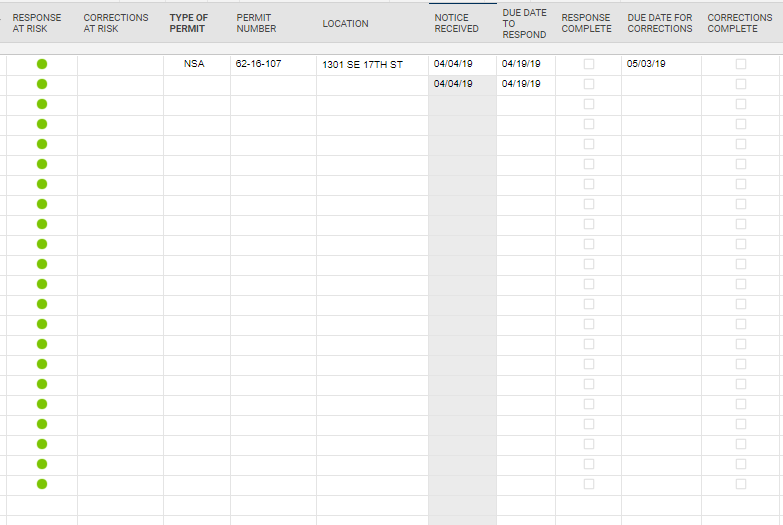# RYG Balls past start date with a complete box

I am trying to automate the ryg ball if i go:

15 days past start date and my complete is blank, red ball

10 days past start date and complete is blank, yellow ball

otherwise green ball.

Any help is appreciated

• You can use the symbols as a dropdown and then the IF statement where the result will be "Red", "Green" or "Yellow". That text as the output of the function will form the colors.

Another thing to think of is that only updates when the sheet is opened and saved again. I've had to integrate with MS Flow to add blank rows just to force a re-calculation for countdown timers and such.

• nested if statments, and(), isblank()

something like

=if(AND(TODAY()-StartDate1>15, isblank(CompleteDate1)),"Red",if(and(Today()-StartDate1>10, isblank(CompleteDate1)), "Yellow","Green"))

• I only get green balls, including in the blanks.

I have attached an example of my sheet• Try this modification which is looking at whether Corrections complete is blank,

=if(AND(TODAY()-[Due Date to respond]@row >15, isblank([Corrections Complete]@row)),"Red", IF(and(Today()-[Due Date to respond]@row>10, isblank([Corrections Complete]@row)), "Yellow","Green"))

Or this modification that is looking for a 0 in the corrections complete.

=if(AND(TODAY()-[Due Date to respond]@row >15, [Corrections Complete]@row = 0),"Red", IF(and(Today()-[Due Date to respond]@row>10, [Corrections Complete]@row=0), "Yellow","Green"))

• =IF(AND(ISTEXT(Location@row), ISBLANK([Completed Column]@row)), IF([Start Date]@row <= TODAY(-15), "Red", IF([Start Date]@row <= TODAY(-10), "Yellow", "Green")))

Give this a whirl...

Mike's looks like it should work as well.• I have worked the formula a little differently.

Red ball if past due date, Yellow if within 5 days of due date, Green if marked complete.

However I want the RYG column to stay blank if the date received column is blank. How do I write that?

Here is my formula so far.

=IF([RESPONSE COMPLETE]1 <> 1, IF(TODAY() - [DUE DATE TO RESPOND]1 > 0, "Red", IF(TODAY() - [DUE DATE TO RESPOND]1 > -5, "Yellow")), IF([RESPONSE COMPLETE]1 = 1, "Green"))

• =IF([RESPONSE COMPLETE]@row= 1, "Green", IF(ISDATE([NOTICE RECEIVED]@row), IF([RESPONSE COMPLETE]@row= 0, IF([DUE DATE TO RESPOND]@row < TODAY(), "Red", IF([DUE DATE TO RESPOND]@row> TODAY(-5), "Yellow")))))

Give this a try.

• Perfect!!

Thank you

• Happy to help!